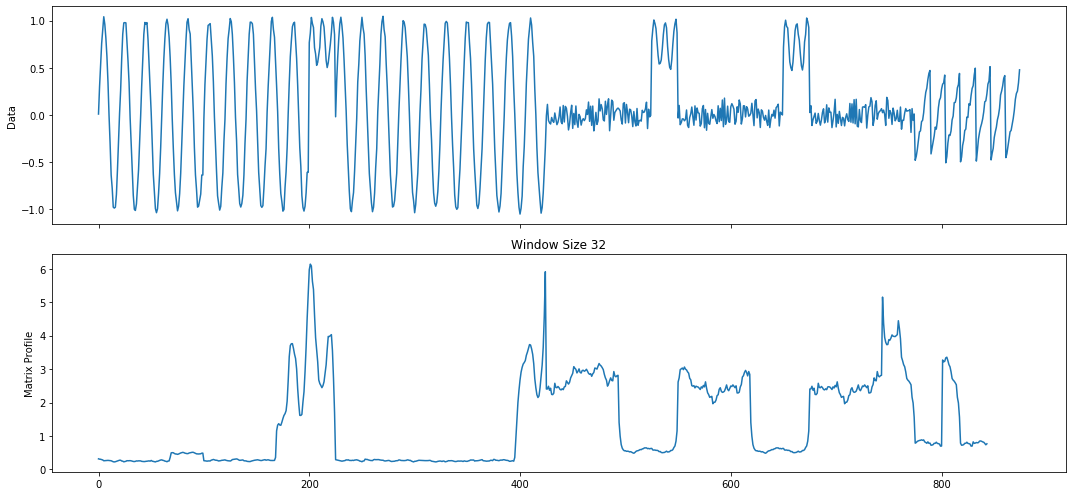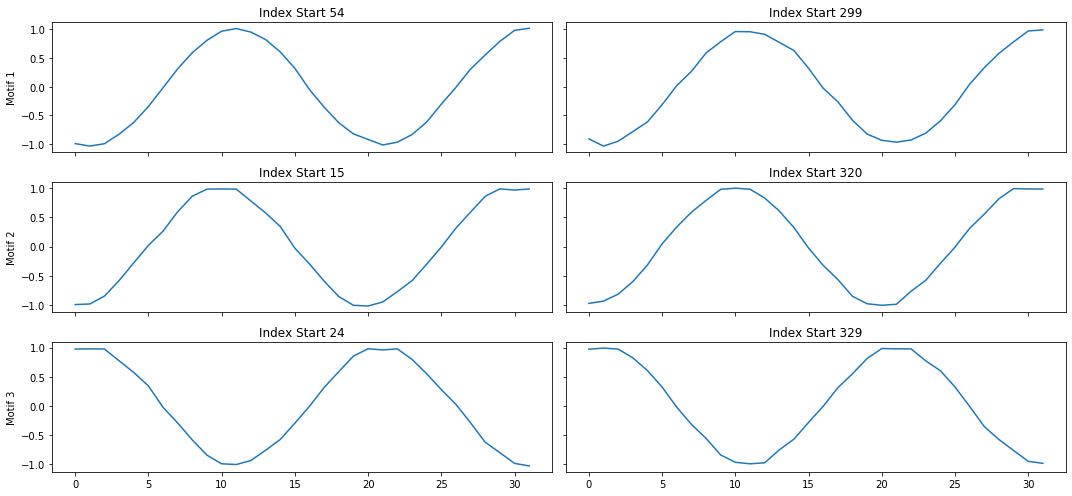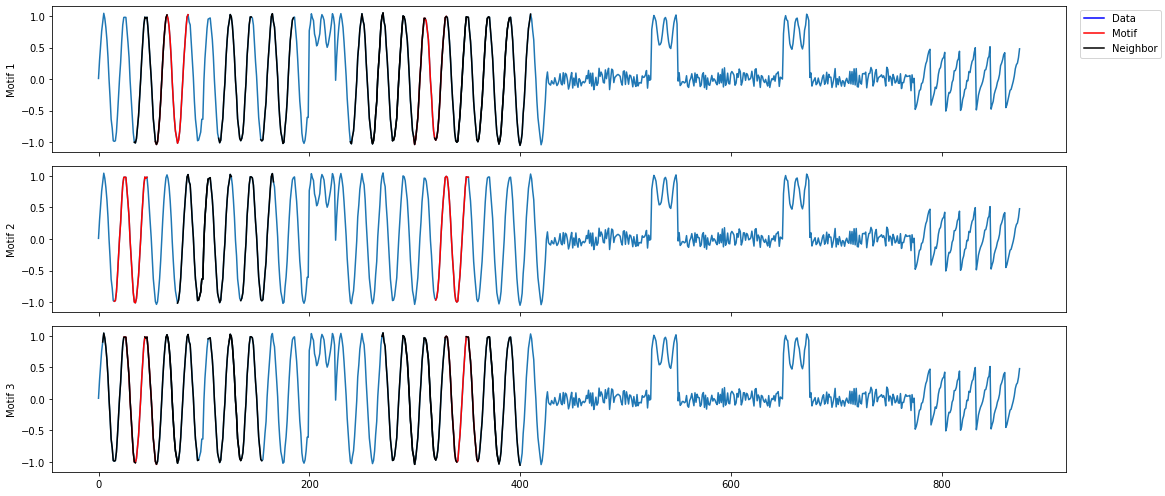# How to Preprocess Your Time Series

## Handling missing data and singular continuous values## Why do we need to pre-process time series data?¶

In most cases, when we feed complete data into compute and analyze functions to compute matrix profiles and analyze time series, all matrix profile algorithms would work well. However, it is inevitable that we have to work with some time series with missing values. In this case, if we don't take steps to handle these missing values, we will get into trouble calculating and analyzing matrix profiles.

The following is an example that will give you an idea of one of the potential problems caused by missing values. To create a time series containing missing data, we insert 'np.nan' and 'np.inf' into the sample dataset used in Quickstart Guide. After that, we try to invoke compute and analyze functions to analyze the time series we created.

In :
```# Import Library
import matrixprofile as mp
import numpy as np
from matplotlib import pyplot as plt
# ignore matplotlib warnings
import warnings
warnings.filterwarnings("ignore")
```
In :
```# Load Data
ts = dataset['data']

# Add missing data to the original time series
ts = np.nan
ts = np.inf

# Compute and analyze the MatrixProfile
profile = mp.compute(ts, windows=32)
print(profile['mp'][:120])

profile, figures = mp.analyze(ts, windows=32)
```
```[ 0.3524921   0.34628064  0.34322172  0.32580627  0.29201275  0.27735623
0.28328524  0.29353866  0.30259366  0.30245875  0.29325783  0.28670776
0.2891161   0.27326822  0.26599104  0.26778939  0.27401618  0.28752641
0.2735069   0.29383559  0.3013937   0.29553578  0.27517326  0.29707322
0.29201275  0.27735623  0.28328524  0.29353866  0.30259366  0.30245875
0.30284275  0.29632738  0.29299616  0.27326822  0.26599104  0.26778939
0.27401618  0.28752641  0.2735069   0.29383559  0.3013937   0.29553578
0.27517326  0.29707322  0.29634663  0.30099944  0.29869319  0.30675545
0.31605481  0.30861558  0.29325783  0.28670776  0.2891161   0.27925098
0.2760615   0.2826953   0.29079211  0.29488062  0.29928782  0.31948651
0.31036921  0.30410852  0.29756674  0.30407931  0.29960505  0.30823594
0.30305364  0.31327603 11.3137085  11.3137085  11.3137085  11.3137085
11.3137085  11.3137085  11.3137085  11.3137085  11.3137085  11.3137085
11.3137085  11.3137085  11.3137085  11.3137085  11.3137085  11.3137085
11.3137085  11.3137085  11.3137085  11.3137085  11.3137085  11.3137085
11.3137085  11.3137085  11.3137085  11.3137085  11.3137085  11.3137085
11.3137085  11.3137085  11.3137085  11.3137085  11.3137085  11.3137085
11.3137085  11.3137085  11.3137085  11.3137085  11.3137085  11.3137085
11.3137085  11.3137085  11.3137085  11.3137085  11.3137085  11.3137085
11.3137085  11.3137085  11.3137085  11.3137085  11.3137085  11.3137085 ]
```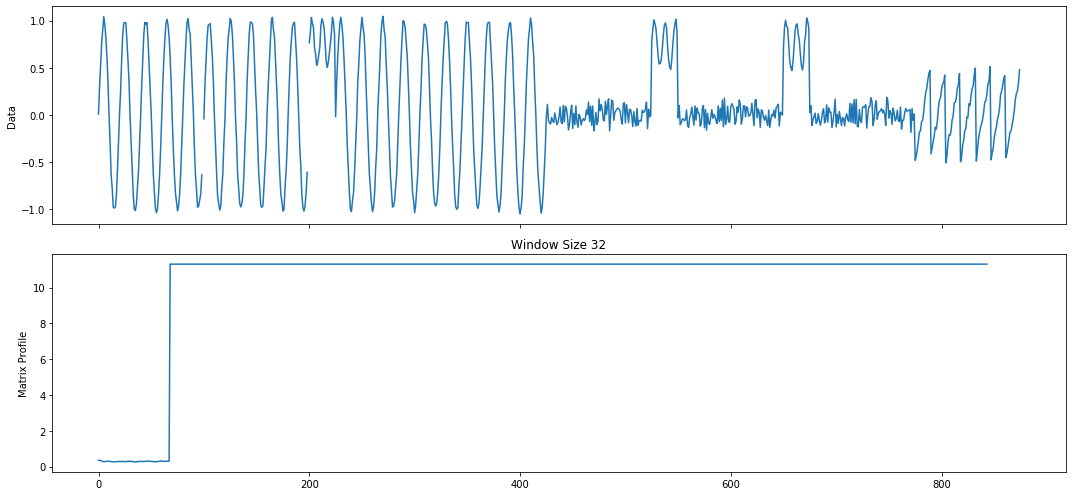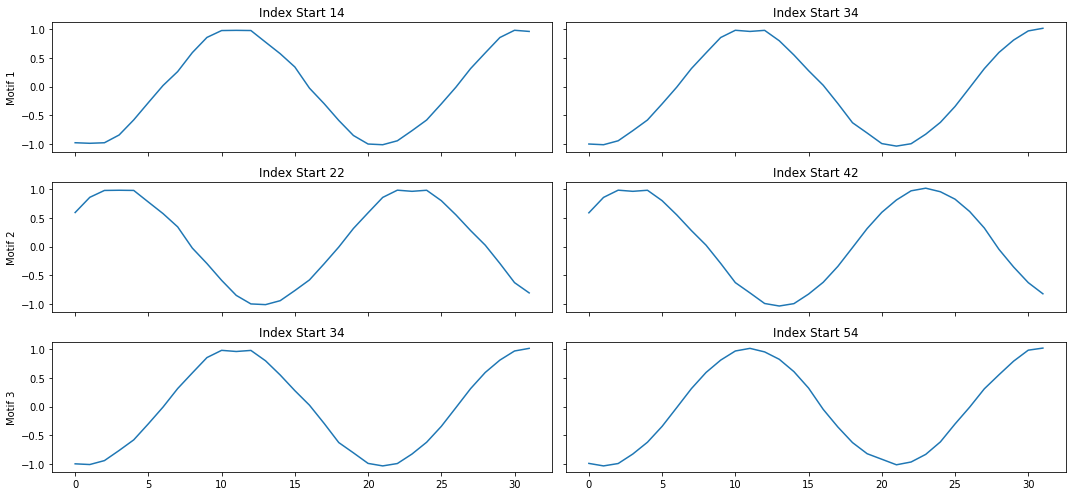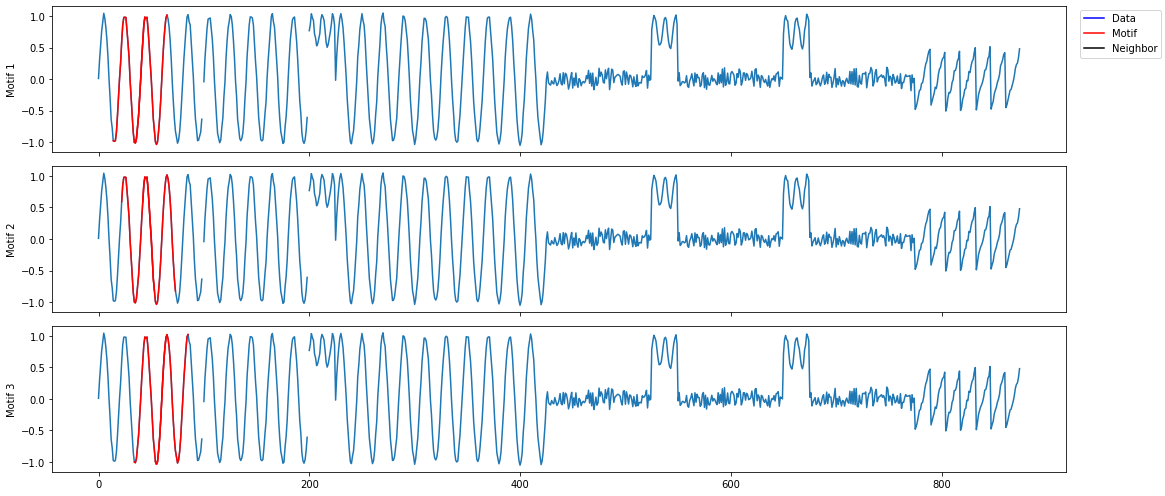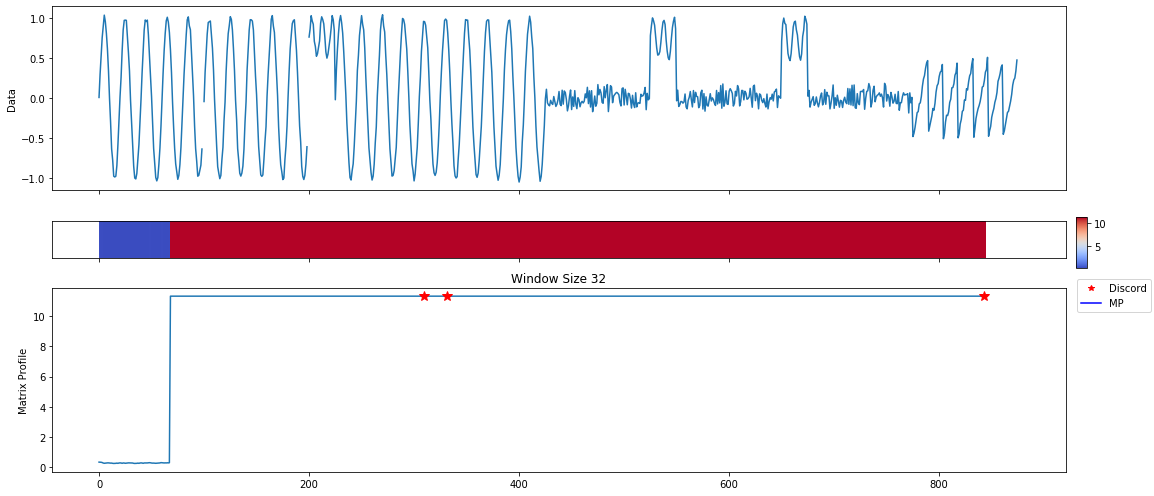We can see from the output and the first two plots that as long as we have missing data somewhere in the time series, the matrix profile values would become constant from the starting position of the subsequence containing the first missing data (which is 68 for this example). You may also see the problems we've run into easily by comparing the above results with the results shown in Quickstart Guide. Clearly, this matrix profile is meaningless, and we are not able to identify the correct motifs and discords based on such a matrix profile.

Therefore, in order to address the potential issues caused by missing data, we introduce a new preprocess module that can help you deal with these issues in some cases.

## Introduce a preprocessing procedure to avoid potential problems with computing and analyzing MatrixProfiles¶

### Enable preprocessing in compute and analyze functions¶

Compute and analyze methods now accept a new parameter preprocessing_kwargs of type dict to enable preprocessing. A valid preprocessing_kwargs has the following data structure:

In [ ]:
```{
# The window size of type int to compute the mean/median/minimum/
# maximum value. The default is 4.
'window': 4,

# A string indicating the data imputation method, which should be
#'mean', 'median', 'min' or 'max'. The default is 'mean'.
'method': 'mean',

# A string indicating the data imputation direction, which should be
# 'forward', 'fwd', 'f', 'backward', 'bwd', 'b'. If the direction is
# forward, we use previous data for imputation; if the direction is
# backward, we use subsequent data for imputation.
# The defualt is 'forward'.
'direction': 'forward',

# A boolean value indicating whether noise needs to be added into the
# time series. The default is True.
}
```

After defining the preprocessing_kwargs, we can enable the preprocessing procedures to impute the missing data in time series. Let's go back to the time series we just created in the previous section and see what will happen to the results after passing in the parameter preprocessing_kwargs to the compute and analyze methods.

In :
```preprocessing_kwargs = {
'window': 3,
'impute_method': 'max',
'impute_direction': 'forward',
}

# Compute and analyze the MatrixProfile
profile = mp.compute(ts, windows=32, preprocessing_kwargs=preprocessing_kwargs)
print(profile['mp'][:120])

profile, figures = mp.analyze(ts, windows=32, preprocessing_kwargs=preprocessing_kwargs)
```
```[0.31603817 0.30619706 0.30307844 0.29103086 0.28242058 0.25885435
0.26178487 0.26298512 0.26445091 0.26975771 0.26682685 0.26496043
0.25899444 0.24459888 0.22846792 0.22436775 0.22991552 0.24116657
0.25282172 0.26204652 0.27500821 0.27407045 0.25155684 0.24706898
0.2269333  0.23478714 0.24319875 0.2605994  0.25807781 0.26123489
0.26173181 0.25653847 0.24557557 0.23782793 0.23510445 0.24067795
0.25391608 0.25052436 0.25582849 0.25490393 0.25472432 0.24074507
0.23708734 0.23448261 0.23528765 0.24191318 0.24499901 0.25154246
0.25539121 0.24605709 0.2675543  0.24639792 0.24022651 0.23150459
0.22415014 0.23995551 0.24344299 0.25386194 0.26955302 0.28032537
0.28508362 0.27185279 0.26480609 0.24829441 0.24285863 0.22918342
0.25004307 0.25345431 0.35849266 0.49543647 0.50105383 0.49806007
0.47591045 0.46205333 0.46005262 0.45048287 0.46030838 0.47773818
0.4914458  0.50081939 0.51002046 0.50171878 0.48617977 0.47886237
0.47216093 0.47351297 0.49080934 0.49704537 0.50348166 0.51198681
0.51040354 0.49513524 0.4804329  0.46575567 0.46207885 0.46116633
0.46325885 0.46738552 0.4846308  0.48682524 0.26038312 0.25710507
0.25297561 0.24618272 0.25279359 0.25406379 0.25319811 0.27700531
0.28500901 0.29759541 0.28651966 0.2757552  0.27382363 0.2661272
0.25210901 0.25765073 0.26259892 0.27251315 0.27467799 0.28032537]
```